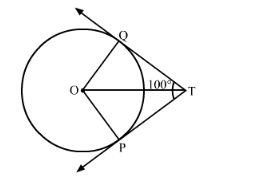# Two tangents TP and TQ are drawn from an external point T to a circle with centre O

Question:

Two tangents TP and TQ are drawn from an external point T to a circle with centre O as shown in Fig. 10.73. If they are inclined to each other at an angle of 100°, then what is the value of ∠POQ?Solution:

Consider the quadrilateral OPTQ. It is given that PTQ = 100°.

From the property of the tangent we know that the tangent will always be perpendicular to the radius at the point of contact. Therefore we have,We know that the sum of all angles of a quadrilateral will always be equal to 360°.

Therefore,++Let us substitute the values of all the known angles. We have,

$100^{\circ}+90^{\circ}+90^{\circ}+\angle \mathrm{POQ}=360^{\circ}$

$280+\angle \mathrm{POQ}=360^{\circ}$

$\angle \mathrm{POQ}=80^{\circ}$

Therefore, the value of angle POQ is 80°.